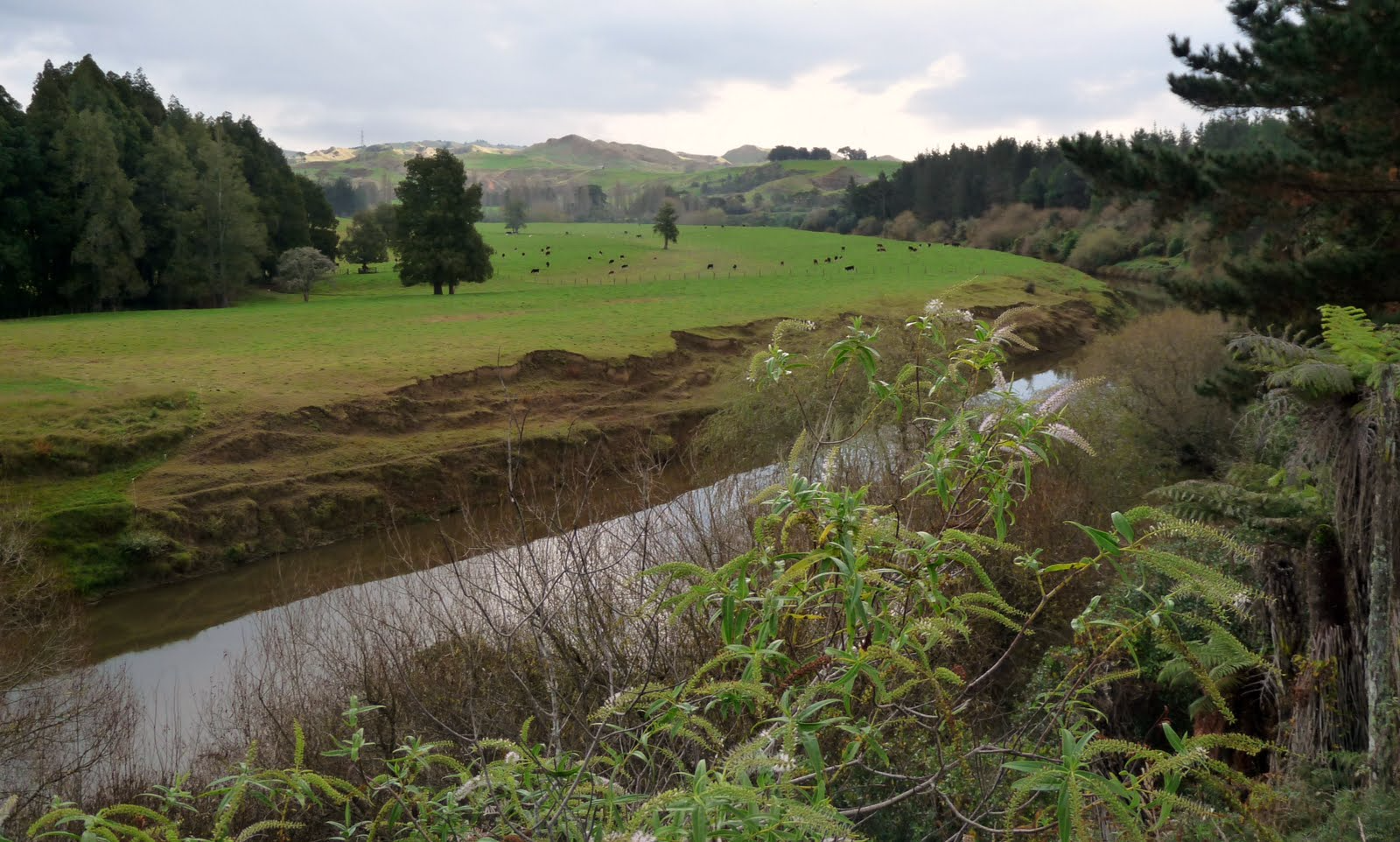# Fixed Perimeter

Topic:
PerimeterThis land is yours and you have 200m of fencing to make an enclosed farming area. How do you construct your farm so that you make the largest farming area possible?

What is the equation for the Area of the farm?

What is the equation for the Perimeter of the farm?

For which equation are you trying to find the largest dimension?

By combining the Area and Perimeter equations so that you are optimising the correct one, what equation did you derive?

## In regards to the graph of A(W) above...

Explore the graph and move it around. Answer the following: Does it have a maximum or minimum? Does this make sense? Why or why not? In the context of this problem what does the y-axis represent? x-axis? What does the max/min mean in the context of this problem, what information does it give us?